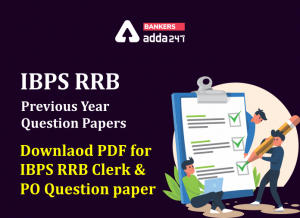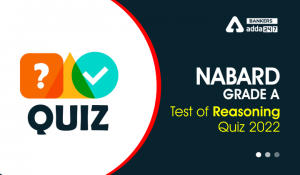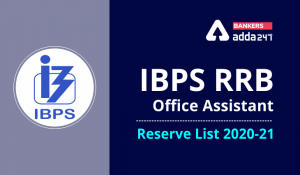Latest Banking jobs   »   Reasoning Ability Quiz For IBPS RRB...

# Reasoning Ability Quiz For IBPS RRB PO, Clerk Prelims 2021- 27th May

Direction (1-5): In each of the questions below are given some statements followed by some conclusions. You have to take the given statements to be true even if they seem to be at variance with commonly known facts. Read all the conclusions and then decide which of the given conclusions logically follows from the given statements disregarding commonly known facts.
(a) If only conclusion I follows.
(b) If only conclusion II follows.
(c) If either conclusion I or II follows.
(d) If neither conclusion I nor II follows.
(e) If both conclusions I and II follow.

Q1. Statements: P > Y ≤ H ≥ U > T = N
Conclusions: I. P > U II. N < H

Q2. Statements: A ≥ S > D = C ≥ V > F > G ≥ H
Conclusions: I. V < D II. H ≤ V

Q3. Statements: F > G ≥ T ≥ H > Y, T ≤ L = J < K
Conclusions: I. J > H II. J = H

Q4. Statements: B > N ≥ M = K > L, W ≤ E = B, Q ≥ W > A
Conclusions: I. W > M II. Q ≤ K

Q5. Statements: C > V = B > N ≥ M, K ≤ L = O ≤ P < V
Conclusions: I. O < C II. B > L

Direction (6-10): In each of the questions below are given some statements followed by two conclusions. You have to take the given statements to be true even if they seem to be at variance with commonly known facts. Read all the conclusions and then decide which of the given conclusions logically follows from the given statements disregarding commonly known facts.
(a) If only conclusion I follows.
(b) If only conclusion II follows.
(c) If either conclusion I or II follows.
(d) If neither conclusion I nor II follows.
(e) If both conclusions I and II follow.

Q6. Statements: P>S≥T; T>Q<R; R=N
Conclusion I: P>N II: R≥S

Q7. Statements: A>B<C=D≥E≥ G<F
Conclusion I: B>F II: C≥G

Q8. Statements: J>K=L; L>N>M; M<A
Conclusion I: J>M II: A>J

Q9. Statements: B=Y≥X>V; V<K>Q; Q=C
Conclusion I: B>V II: C≤X

Q10. Statements: Z>V<N=M≥E≥B<C
Conclusion I: B>V II: Z≥E

Direction (11-15): In each of the questions below are given some statements followed by some conclusions. You have to take the given statements to be true even if they seem to be at variance with commonly known facts. Read all the conclusions and then decide which of the given conclusions logically follows from the given statements disregarding commonly known facts.
(a) If only conclusion I follows.
(b) If only conclusion II follows.
(c) If either conclusion I or II follows.
(d) If neither conclusion I nor II follows.
(e) If both conclusions I and II follow.

Q11. Statements: P ≥H >T= Y >Q ≤N >E <F
Conclusions: I. P > N II. F< H

Q12. Statements: Z ≥S >N = C ≥ V; V>J >L ≥ H
Conclusions: I. L< N II. H ≤ V

Q13. Statements: F > S > T ≥ H > D; F ≤ L = J < G
Conclusions: I. J > H II. S < G

Q14. Statements: M > N ≥ R = T > L; M ≤ E < B; L < W > A
Conclusions: I. E > T II. A ≤ M

Q15. Statements: M > K = L> N ≥ A; M ≤ L = O ≤ P < V
Conclusions: I. N < P II. M ≤P

Practice More Questions of Reasoning for Competitive Exams:

###### Study Plan for IBPS RRB PO/Clerk Prelims 2021

Solutions

S1. Ans. (b)
Sol. I. P > U (False) II. N < H (True)

S2. Ans. (d)
Sol. I. V < D (False) II. H ≤ V (False)

S3. Ans. (c)
Sol. I. J > H (False) II. J = H (False)

S4. Ans. (d)
Sol. I. W > M (False) II. Q ≤ K (False)

S5. Ans. (e)
Sol. I. O < C (True) II. B > L (True)

S6. Ans. (d)
Sol. I: P>N (False) II: R≥S (False)

S7. Ans. (b)
Sol. I: B>F (False) II: C≥G (True)

S8. Ans. (a)
Sol. I: J>M (True) II: A>J(False)

S9. Ans. (a)
Sol. I: B>V (True) II: C≤X (False)

S10. Ans. (d)
Sol. I: B>V (False) II: Z≥E(False)

S11. Ans. (d)
Sol. I. P >N(False) II. F <H(False)

S12. Ans. (a)
Sol. I. L <N(True) II. H ≤ V(False)

S13. Ans. (e)
Sol. I. J >H(True) II. S <G(True)

S14. Ans. (a)
Sol. I. E >T(True) II. A ≤ M(False)

S15. Ans. (e)
Sol. I. N <P(True) II. M ≤ P(True)#### Congratulations!Incorrect details? Fill the form again here

•IBPS RRB Previous Year Question Paper PD...
•Reasoning Ability Quiz For IBPS RRB PO C...
•Reasoning Ability Quiz For IBPS Clerk Pr...
•Reasoning Ability Quiz For NABARD Grade ...
•Reasoning Ability Quiz For IBPS Clerk Pr...
•IBPS RRB Clerk 2nd Reserve List 2022 Out...